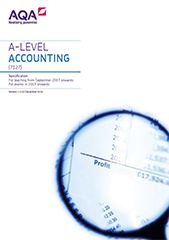# Annex: quantitative skills in accounting

In order to develop their skills, knowledge and understanding in accounting, students need to have acquired competence in the quantitative skills that are relevant to the subject content and which are applied in the context of an accounting A-level, including:

• calculate, use and understand ratios and fractions
• calculate, use and understand percentages and percentage changes
• calculate cost, revenue, profit and break-even
• calculate investment appraisal outcomes and interpret results
• calculate and apply payback and net present value including the use of discounting techniques
• calculate and interpret variances
• calculate total product cost and selling price using activity based costing and absorption costing
• interpret, apply and analyse information in written, graphical and numerical forms
• use and interpret quantitative and non-quantitative information in order to make decisions.

A minimum of 20% of the overall A-level marks will be attributed to the assessment of quantitative skills at a level of demand which is not lower than that which is expected of learners in assessments for the higher tier in a GCSE qualification in mathematics. These skills may be assessed across the assessment objectives.